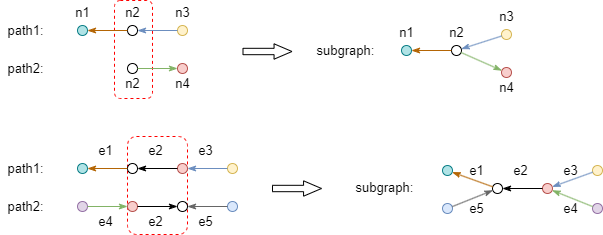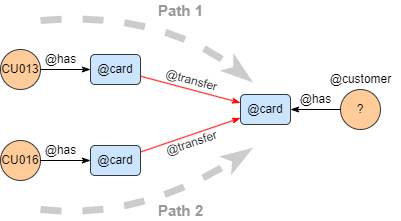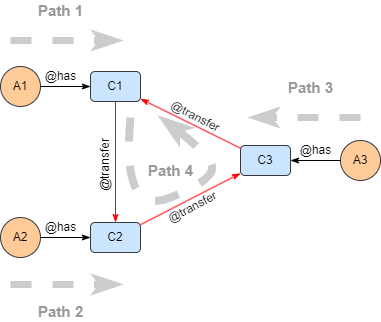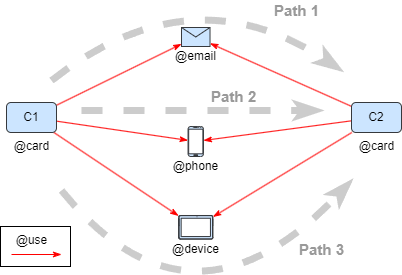# Change Nickname

Current Nickname:
Search
v4.0
v4.0

# Subgraph Template

## Command, parameters

Subgraph template query is a filtering and querying against a subgraph that is made by multiple path templates. The relationship between the template paths is indicated by calling the `custom alias` defined in the previous template paths, such as paths intersect at a certain node, paths have common edges, and so on.Syntax:

• Command: `graph()`
• Parameter: Subgraph made by path templates (Note: Write in the command)
• Statement Alias: Not supported

## Single Relational Alias

Example: as the path structure shown in the graph below, find the customers who received transfer from Customer CU013 and CU016, return with all properties.``````graph([
n({_id == "CU013"}).re().n({@card}).re({@transfer}).n({@card} as n).le().n({@customer} as c),
n({_id == "CU016"}).re().n({@card}).re({@transfer}).n(n)
])
return c{*}
``````

Analysis: this subraph uses Node Alias n to relate the two paths with each other.

## Multiple Relational Alias

EExample: as the path structure shown in the graph below, find the circular paths: 3 distinct customer's cards perform circular transfer in a single direction, return customers in group.``````graph([
n({@customer} as A1).re().n({@card} as C1),
n({@customer && _uuid < A1._uuid} as A2).re().n({@card} as C2),
n({@customer && _uuid < A2._uuid} as A3).re().n({@card} as C3),
n(C1).re().n(C2).re().n(C3).re().n(C1)
])
return A1{*}, A2{*}, A3{*}
``````

Analysis: this subgraph uses Node Alias C1, C2, and C3, to relate 4 paths to each other.

Example: as the path structure shown in the graph below, find bank card information when 2 distinct cards uses the same email addresses, phone numbers, devices, return with all properties.``````graph([
n({@card} as C1).e().n({@email}).e().n({@card && _uuid > C1._uuid} as C2),
n(C1 as C11).e().n({@phone}).e().n(C2 as C22),
n(C11).e().n({@device}).e().n(C22)
])
return table(C11._id, C22._id)
``````

Analysis: the subgraph uses Node Alias C1, C2 to relate 3 paths to each other. If an alias is to be referenced for multiple times, it needs to be redefined each time after it is referenced: C1 is redefined as C11, C2 as C22 in the example above.